Ampere's law to calculate the B field from a balanced three-phase system

I am a little confused when I try to use Ampere's law to calculate the B field from a balanced three-phase system.

Consider the following, shown in the picture below:
Lets say I want to calculate the value of B at a distance r (radius of my Amperian loop), the value of r is big enough so that the loop encircles all three conductors.

From Ampere's law we have:
$\oint_{c}\vec{B}\cdot d\vec{l} = \mu\times I_{enclosed}$

However, since the sum of the three currents = 0, i.e. net current out of the surface encircled by the curve C is equal to 0, indicates that the value of B at point r is also 0.

So what am I doing wrong, how should I apply Ampere's law to get the correct result?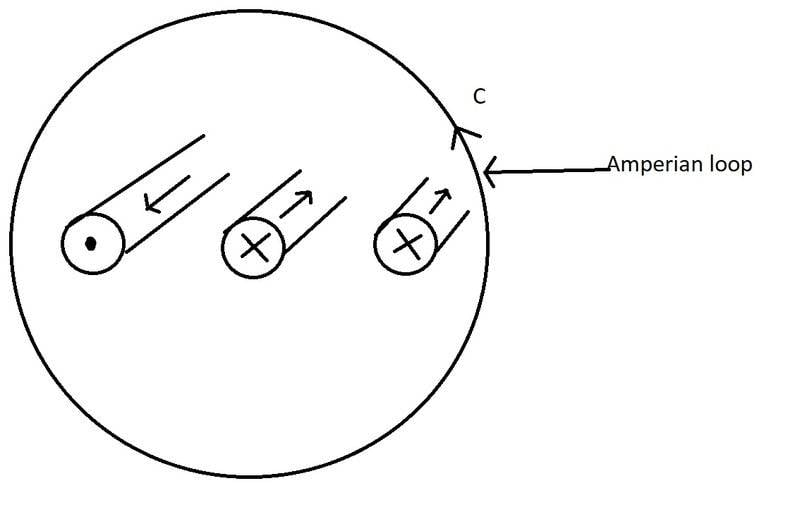Homework Helper
Gold Member
As I understand it, the 3-phase current has ## I_i=I_o cos(\omega t+\phi_i) ## where the phases are 120 degrees apart, and so the 3 sinusoidal currents add to zero. If I'm not mistaken, you are correct in your answer that ## B =0 ##. Let's see if anyone else will concur.

jim hardy
Gold Member
Dearly Missed
Ampere's Law Rules !

If you place a clamp-around ammeter around those three conductors it will indeed indicate zero , for the reason Charles Link stated.

Same is true for a single phase two wire circuit where you encircle the supply and return conductors.

That's how the GFCI fault detector receptacles that ought to be in in your kitchen and bathroom work.

http://www.ecmweb.com/basics/how-gfcis-work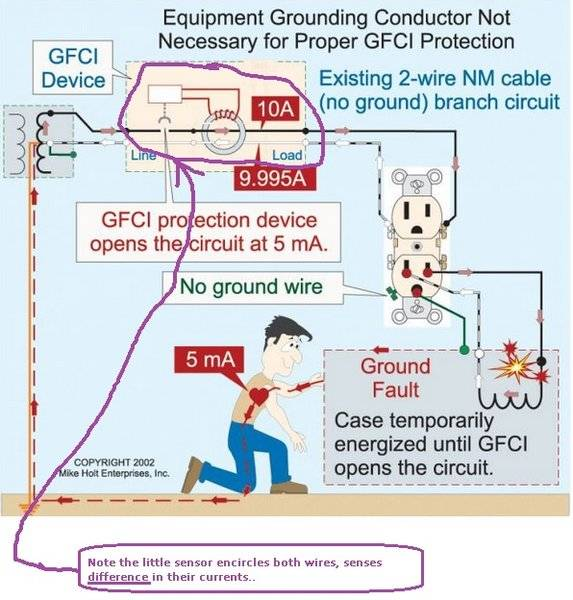Now there might be some B at individual points out there but integrating B around a loop will get you zero, or I enclosed.

For B at an individual point try a Biot-Savart calculation to each conductor ?
EDIT @OliskaP
I think i made a boo-boo. You won't have to invoke Biot after all see two posts down.

Last edited:
•Asymptotic, OliskaP, dlgoff and 1 other person
Thanks for the replies. I looked at a table which showed typical values of B at a distance x from a 420 kV transmission line, and they were not zero. So if I would want to calculate the value of B below the transmission line at ground level, I would have to use Biot-Savart equation on each conductor and sum them up?

jim hardy
Gold Member
Dearly Missed
Thanks for the replies. I looked at a table which showed typical values of B at a distance x from a 420 kV transmission line, and they were not zero. So if I would want to calculate the value of B below the transmission line at ground level, I would have to use Biot-Savart equation on each conductor and sum them up?
EDITED I believe that's how to approach it. Whole is equal to the sum of its parts.
see next post

Have you a link to that table?

old jim

Last edited:
jim hardy
Gold Member
Dearly Missed
Hmmmmmm

Hmmmm after some further looking ,
perhaps you should try this Ampere's law for each individual conductor and add them , considering geometry of the wires and observer?
from hyperphysics http://hyperphysics.phy-astr.gsu.edu/hbase/magnetic/magcur.html#c3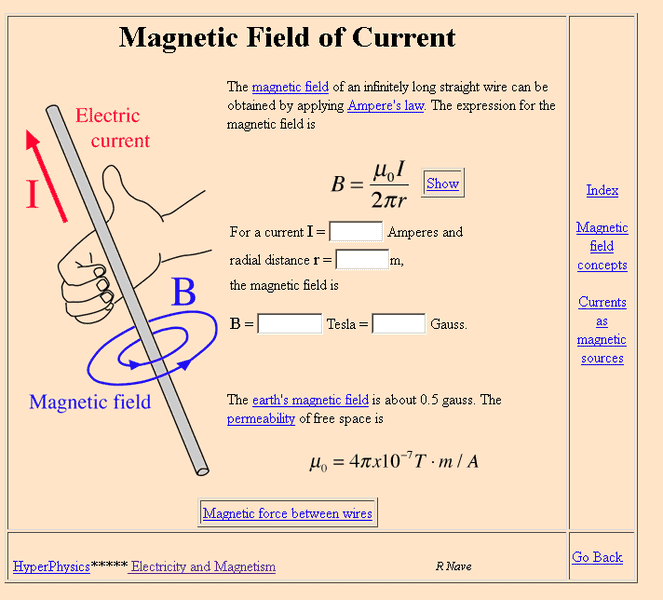The farther from the wires you get the more nearly the individual fields will cancel , as the difference in their r's becomes insignificant.

I found this tutorial from a UK utility . It's well written and straightforward.

and that's their approach.

old jim

Last edited:
•Asymptotic, Charles Link, OliskaP and 1 other person
I will have a look at the pdf from the UK utility company later when I am home.

Have you a link to that table?

The table is in a compendium written in Norwegian by a professor at the Norwegian university of science and technology, and his source is the Norwegian radiation protection authority, this one is also in Norwegian.

From the source in norwegian, they consider a value of 0.4 μT to be the safe limit for long term exposure. On page 5 they state that for a 420 kV line you would in some cases need to be 80-100 meters away from the transmission line for the value of B to be 0.4 μT or less.

Source (in norwegian): http://www.nrpa.no/publikasjon/bolig-naer-hoeyspenningsanlegg.pdf

Another source in english (http://www.who.int/peh-emf/project/mapnatreps/nznrl_emfbooklet2008.pdf) on page 6, states that the magnetic field 40 meters from a high voltage transmission line to be around 0.1-1 μT

•jim hardy and Asymptotic
EDIT @OliskaP
I think i made a boo-boo. You won't have to invoke Biot after all see two posts down.

Thanks Jim, and after reading the pdf you linked in post #6, it became clear.
First we consider one conductor and calculate the value of B at point P, then we consider the other two conductors individually and add the values of B from the three conductors to get the value of B at point P.

•jim hardy
By the way, lets say I never saw that table stating that the value of B is different from zero at point P. So without knowing about thetable, I would apply Ampere's law as shown in the picture from post #1, and concluded that the value of B to be zero for every point in space that lies on the Amperian loop.

How am I supposed to know that I have to use Ampere's law on each individual conductor and not all three at the same time? Is the equation telling me something that I have not understood?

Homework Helper
Gold Member
The way I understand it, if the 3 phases are precisely 120 degrees apart and the currents are equal in amplitude, if you are far enough away from the 3 conductors that you are equidistant from the 3 conductors, the magnetic field will be zero because the 3 currents will add to zero, (using a vector phasor diagram or by using trigonometric identities). If you are close to the conductors, the wires are not all perfectly equidistant from you because they need to be separated by a finite distance and a magnetic field results.

•OliskaP, jim hardy and anorlunda
jim hardy
Gold Member
Dearly Missed
So without knowing about thetable, I would apply Ampere's law as shown in the picture from post #1, and concluded that the value of B to be zero for every point in space that lies on the Amperian loop.

Ampere's law describes a close loop path. That's what the little circle in the middle of the integral sign is for. Note in that first picture you take the dot product around the path so answer is a scalar.

http://hyperphysics.phy-astr.gsu.edu/hbase/magnetic/amplaw.html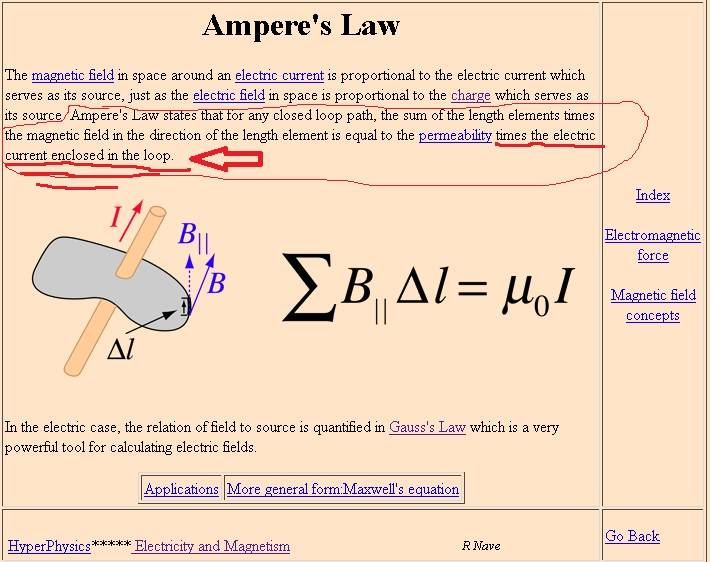If that loop encloses some current there'll be a field surrounding it.
So i think the short answer to your question is you have to pick your loop carefully so that it encloses the current of interest.

http://hyperphysics.phy-astr.gsu.edu/hbase/magnetic/magcur.html#c3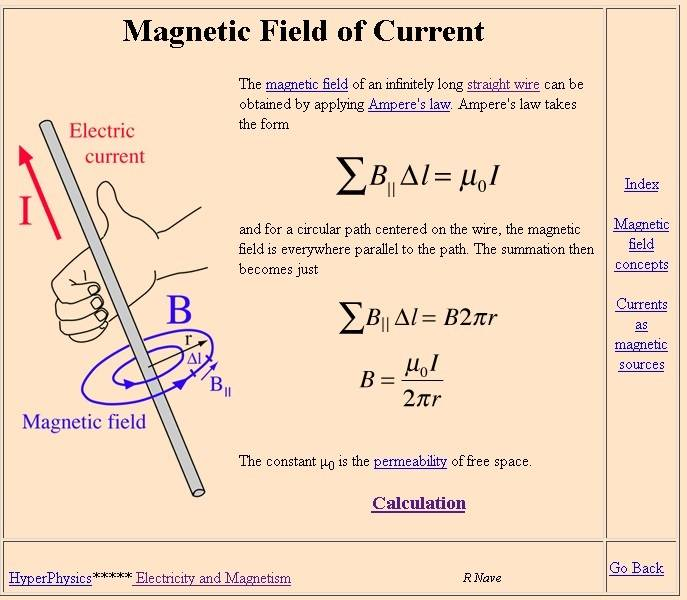once we pick the path to enclose nonzero current we'll get nonzero flux for the conductor that we encircled ! .

More subtle is this - observe second equation is not a summation or an integration, but the result of one.
It gives you the magnitude of flux at any point on the closed loop path. That path is a circle of radius r.
You'll have to ascribe to that flux a direction, along the path of that circle, in order to make it into a vector..

Biot-Savart does that for you, its multiplication is a vector cross product. In my youth i loved to work vector cross products, nowadays i seem to just talk about them. Cross product gives you a vector, dot product just a scalar..

How am I supposed to know that I have to use Ampere's law on each individual conductor and not all three at the same time? Is the equation telling me something that I have not understood?

Hmmmm... For old troubleshooters it quickly becomes instinctive that a clamp around meter around all the wires reads zero but it'll read individual wires just fine.
.For a student perhaps it's best to work the other way

The form of Ampere's law that you chose
∮c⃗B⋅d⃗l=μ×Ienclosed
dictates you sum the fluxes at every point around the Amperian loop . But you're not interested in the total of all the points, just one of them.
Flux at a single point is given by the second form , but only its magnitude since it's a scalar.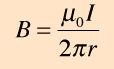Standing under your power lines the magnetic field from each wire will have a direction too, along the circular path.

At any point below your power lines , you are not equidistant from all three wires. So which I and r will you use ? You have to use all of them and superposition,
calculate each break it into X and Y components then add , like in that tutorial .

Any help ?

old jim

Last edited:
•anorlunda and OliskaP
Thanks, both of you, I really appreciate the help. Things are much clearer now.

•anorlunda
Staff Emeritus
@jim hardy is my favorite hands on guy, but he could have earned a living as an analyst too. His answer in #11 is spot on.

But let me explain how you can combine common sense with analytical tools. You could have a three phase system withe one phase right next to you and the other two phases on the far side of the galaxy. Common sense tells you that the two remote phases should play no practical role on what you sense locally. Therefore, if you applied Amperes law to the singe local phase, or if you applied it cottectly to all three phases you should get the same answer. The key is the word correctly. Ignoring the location of the three phases as in the OP gives the answer zero. That's rediculous so it can't be correct. Doing it one phase at a time and superimposing the results gives you the right answer.

If you want to be an engineer, as you learn of analytical tools like Amperes Law, do not forget your common sense. Unless you are dealing with truly weird stuff like QM or GR, the results from the powerful analytical tools like Maxwells Equations always agree with common sense. When they seem to disagree, as in this thread's OP, it is a clue that you're doing it wrong.

•jim hardy
Homework Helper
Gold Member
@jim hardy is my favorite hands on guy, but he could have earned a living as an analyst too. His answer in #11 is spot on.

But let me explain how you can combine common sense with analytical tools. You could have a three phase system withe one phase right next to you and the other two phases on the far side of the galaxy. Common sense tells you that the two remote phases should play no practical role on what you sense locally. Therefore, if you applied Amperes law to the singe local phase, or if you applied it cottectly to all three phases you should get the same answer. The key is the word correctly. Ignoring the location of the three phases as in the OP gives the answer zero. That's rediculous so it can't be correct. Doing it one phase at a time and superimposing the results gives you the right answer.

If you want to be an engineer, as you learn of analytical tools like Amperes Law, do not forget your common sense. Unless you are dealing with truly weird stuff like QM or GR, the results from the powerful analytical tools like Maxwells Equations always agree with common sense. When they seem to disagree, as in this thread's OP, it is a clue that you're doing it wrong.
What isn't completely clear on the OP's post, (and this is for the OP @OliskaP ), is if this is a posting where it is a very simple question looking for a simple answer like you might get in an introductory physics class, or if the OP is actually looking for a more thorough analysis like @jim hardy and @anorlunda have provided. ## \\ ## If the question was presented as a very introductory physics problem, the answer would be, in a very simplified (and really not completely correct) form, equal to zero. In this case the focus would be on applying trigonometric identities and/or a vector phasor diagram to show the answer is zero. ## \\ ## When considering actual power lines in the real world that supply 3-phase power, there is even the question whether it is on large high voltage/high current lines that are part of a power grid, or if it is simply the power supplied as 3-phase power to a large household appliance.

Last edited:
jim hardy
Gold Member
Dearly Missed
Thanks @anorlunda
i'm still not quite satisfied with #11, am trying to figure out how to word it more clearly .
Creative thinking is a process of exaggeration - so thanks for your word picture of an amperian loop encircling Alpha Centauri...
Applying your idea to Oliska's first image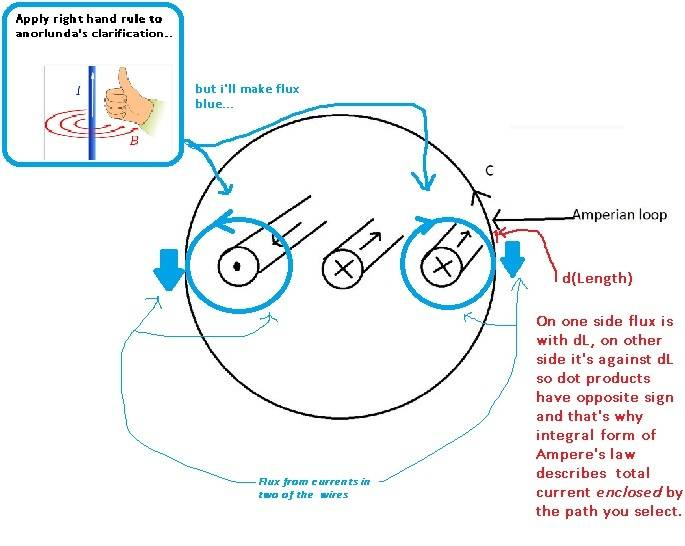The whole is equal to the sum of its parts. Integral form gives the whole, Biot Savart does a proper cross product to give result with direction at any point,

this abbreviated formgives magnitude only of flux at a single point due to a single current at a specific distance.
Fortunately since you draw the diagram you can figure out the direction without the pain of a Biot-Savart integral.

the results from the powerful analytical tools like Maxwells Equations always agree with common sense.
I struggle to make them agree and that's my check&balance . I dont trust an equation until i've done that, for i will invariably mis-apply one that i haven't so checked.

But i plod along.

old jim

•OliskaP
Homework Helper
Gold Member
Thanks @anorlunda
i'm still not quite satisfied with #11, am trying to figure out how to word it more clearly .
Creative thinking is a process of exaggeration - so thanks for your word picture of an amperian loop encircling Alpha Centauri...
Applying your idea to Oliska's first image

View attachment 210133

The whole is equal to the sum of its parts. Integral form gives the whole, Biot Savart does a proper cross product to give result with direction at any point,

this abbreviated form
View attachment 210135
gives magnitude only of flux at a single point due to a single current at a specific distance.
Fortunately since you draw the diagram you can figure out the direction without the pain of a Biot-Savart integral.

I struggle to make them agree and that's my check&balance . I dont trust an equation until i've done that, for i will invariably mis-apply one that i haven't so checked.

But i plod along.

old jim
@jim hardy If you want to make the observation point on the right side of the diagram where the two circles intersect, you need to have the blue circle on the left be a much larger radius, intersecting these two circles at the observation point on the right where the other two circles intersect. Just a simple input that might help clarify it considerably.

jim hardy
Gold Member
Dearly Missed
If the question was presented as a very introductory physics problem, the answer would be, in a very simplified (and really not completely correct) form, equal to zero. In this case the focus would be on applying trigonometric identities and/or a vector phasor diagram to show the answer is zero. \\ When considering actual power lines in the real world that supply 3-phase power, there is even the question whether it is on large high voltage/high current lines that are part of a power grid, or if it is simply the power supplied as 3-phase power to a large household appliance.

The reason i digressed was Oliska said this, suggesting more than a simple question.
So if I would want to calculate the value of B below the transmission line at ground level,

Can one infer from the closed loop integral form that flux everywhere along the path is zero ? I think not. That was Oliska's trouble i think.

That equation was presented to me in school as H dot dl not B dot dl , and as an analogy to Gauss's law for Efields
It was easier for me to visualize summing the MMF dot dl's than the Flux dot dl's because i was envisioning something familiar - a clamp on ammeter. I actually played with one in lab - when you open the jaws you add reluctance so the reading drops . When you encircle all the wires the reading drops.

as i said, i plod.

old jim

jim hardy
Gold Member
Dearly Missed
@jim hardy If you want to make the observation point on the right side of the diagram where the two circles intersect, you need to have the blue circle on the left be a much larger radius, intersecting these two circles at the observation point on the right where the other two circles intersect. Just a simple input that might help clarify it considerably.

Good thinking. Done.

Note they don't cancel locally, just when integrated over the whole path.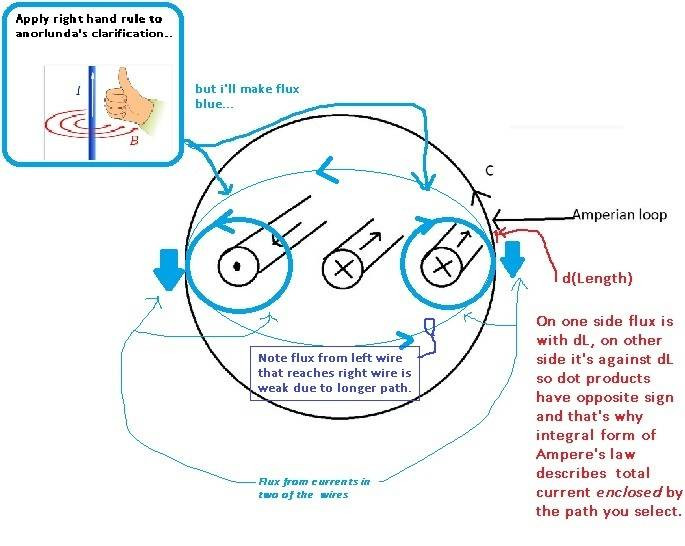So flux at any point along the amperian loop is the sum of its parts, and not necessarily zero.

@Charles Link - Did i get it right ? Is this what you had in mind ?

I could have extended left flux's path way outside the amperian loop - it's a field after all not a discrete line..

Thanks for the suggestion

It is soo very difficult to arrange the little thought steps so as to lead the mind to unambiguous mental image. That's why i quote Lavoisier so often - his introduction to treatise on chemistry belabors that point.
The art of reasoning is nothing more than a language well arranged."
https://web.lemoyne.edu/giunta/EA/LAVPREFann.HTML
He and Asimov were the Jedi Masters.

Homework Helper
Gold Member
Good thinking. Done.

Note they don't cancel locally, just when integrated over the whole path.

View attachment 210136
So flux at any point along the amperian loop is the sum of its parts, and not necessarily zero.

@Charles Link - Did i get it right ? Is this what you had in mind ?
@jim hardy Not quite. The small blue circle on the left needs to be about 4x bigger. ## \\ ## Meanwhile, the algebraic analysis is somewhat simple in that the cosine function will take care of any + and - signs=direction of the current. For the observation point on the right, if the three circles have the same radius,(conductors would have to be very close to each other), we would have ## B=B_o [ \cos(\omega t)+\cos(\omega t+\pi/3)+\cos(\omega t+2 \pi/3)]=0 ##. ( ## \pi/3=120 ## degrees. ## 2\pi/3=240 ## degrees.) ## \\ ## If the radii are different, then we need to write ## B=B_1 \cos(\omega t)+B_2 \cos(\omega t+\pi/3)+B_3 \cos(\omega t +2 \pi/3) ## where ## B_1 ##, ## B_2 ## , and ## B_3 ## are each found by Ampere's law using the radii of the 3 circles in the diagram. This expression can be readily computed, once ## B_1 ## , ## B_2 ## and ## B_3 ## are known, and it will be of the form ## B=B_{total}=B_{to} \cos(\omega t+\phi ) ## for some ## B_{to} ## and ## \phi ##. ## B_{to} ## will not be equal to zero if ## B_1 ##, ## B_2 ## and ## B_3 ## are not all equal.

Last edited:
•OliskaP
jim hardy
Gold Member
Dearly Missed
Not quite. The small blue circle on the left needs to be about 4x bigger.

Okay, and i'll make it green .
How's this?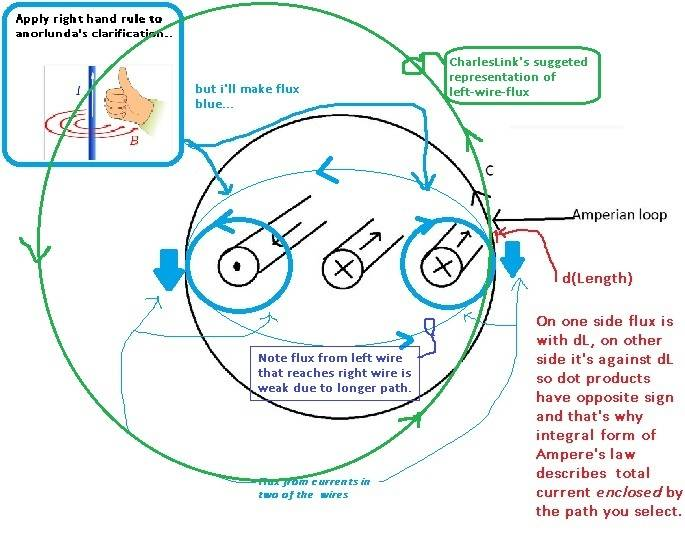it's getting pretty cluttered - i won't try to draw the radii...

I feel a lot better now about our explanation.
Thanks to all who contributed - it's what we do here, share our strengths and work on our weaknesses. In human affairs the whole is a little bit greater than the sum of its parts, isn't it?

old jim

•Homework Helper
Gold Member
Okay, and i'll make it green .
How's this?
View attachment 210139

it's getting pretty cluttered - i won't try to draw the radii...

I feel a lot better now about our explanation.
Thanks to all who contributed - it's what we do here, share our strengths and work on our weaknesses. In human affairs the whole is a little bit greater than the sum of its parts, isn't it?

old jim
@jim hardy The green circle is what I was looking for. Very good. Thanks. :) :) ## \\ ## And for each of these 3 circles with radius ## R_i ##, we have Ampere's law which gives ## B_i \, (2 \pi \, R_i)=\mu_o I ##, so we see that ## B_i =\mu_o I/(2 \pi R_i ) ## is different for each.

Last edited:
•jim hardy
anorlunda
Staff Emeritus
Good job guys.

Now, the picture shows the setup for a double circuit transmission tower. Two three phase circuits. If I present it as a fact that the capacity is less than twice the capacity of one circuit (and staying with the thoughts of this thread) what does that tell you?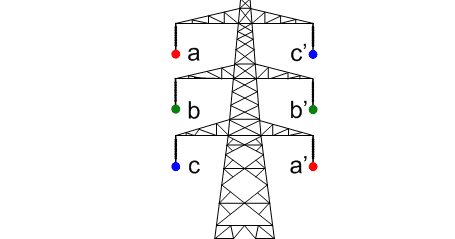•Asymptotic, jim hardy and Charles Link
Homework Helper
Gold Member
Good job guys.

Now, the picture shows the setup for a double circuit transmission tower. Two three phase circuits. If I present it as a fact that the capacity is less than twice the capacity of one circuit (and staying with the thoughts of this thread) what does that tell you?

View attachment 210147
@anorlunda Very good. If I interpret what you are saying correctly: Are you saying that the magnetic fields from one wire cause magnetic fields at the other wires (cables), so that it is impossible to run as much current down each phase of a 3-phase system as you can down a single wire of single phase system without getting power losses caused by these magnetic fields? ## \\ ## Editing: Looking at the diagram and description more closely, I see it is a dual 3-phase system. Thereby, it is more complicated than a single 3-phase system, but basically the magnetic fields from the two different systems will interfere with each other, so you can't get twice the power through this system that you can from a single 3-phase system.

Last edited:
•Asymptotic and anorlunda
Thanks again.

I played around a little, and tried to calculate the magnitude of the resultant $\vec{B}$ at point P and got the figure below.
What do you guys think, looks reasonable? I might have done some errors, I did this in a rush. The interesting point is that the magnitude of B is zero some times.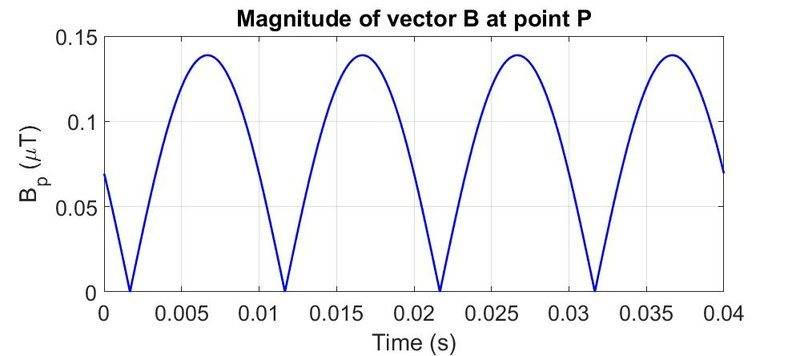Here is my schematic showing the different lengths etc. I used however 100 m as height h and x = 100 m as the distance between the right conductor and the observation point.

Other information:
Balanced three phase system, Irms = 1000 A, conductor diameter = 144 mm, distance d between conductors = 6 m.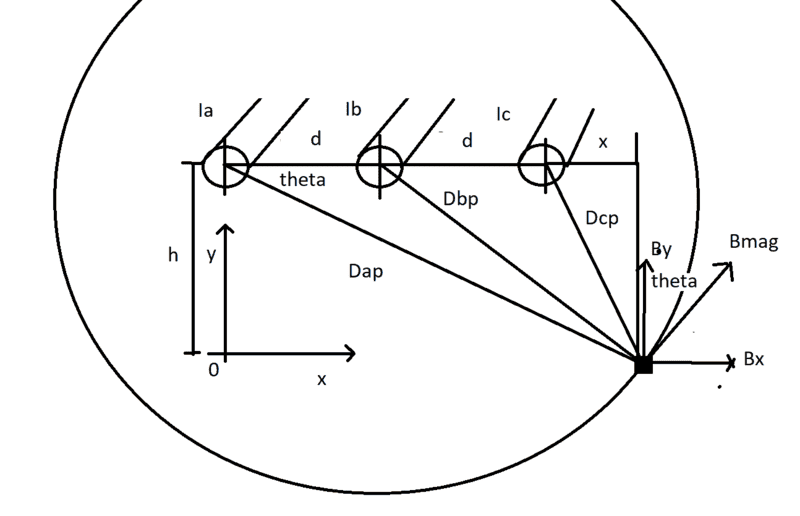CODE USED TO GENERATE FIGURE 1 BASED ON FIGURE 2:
Code:
clc;
clear;
close all;

u0 = 4*pi*1e-7; %permeability of free space
r = 114/1000/2; %radius of conductor (conductor diameter 114 mm)
d = 6;      %Distance between conductors in meters
h = 100;    %Height distance between point P and center of conductor
x = 100;    %Horizontal distance between right conductor and point P

Time = 0;
EndTime = 2/50;
StepTime = 1e-6;

ReportCounter=0;
ReportInterval=1;
Counter=ReportInterval;

%Distances between conductor n and point p
Dap = sqrt((d+d+x)^2+h^2);
Dbp = sqrt((d+x)^2+h^2);
Dcp = sqrt(x^2+h^2);

%Angels used to get x and y component for vector B
Sintheta_a = h/(sqrt(h^2+(d+d+x)^2));
Costheta_a = (d+d+x)/(sqrt((d+d+x)^2+h^2));

Sintheta_b = h/(sqrt(h^2+(d+x)^2));
Costheta_b = (d+x)/(sqrt((d+x)^2+h^2));

Sintheta_c = h/(sqrt(h^2+x^2));
Costheta_c = x/(sqrt(x^2+h^2));

%Time loop to calculate each value of B between time = 0 and time = 2/50 s
while Time<EndTime

%Three-phase currents at time = t
Ia = 1000*sqrt(2)*cos(2*pi*50*Time);
Ib = 1000*sqrt(2)*cos(2*pi*50*Time+120*pi/180);
Ic = 1000*sqrt(2)*cos(2*pi*50*Time+240*pi/180);

%B magnitude
Bap = u0*Ia/2/pi/Dap;
Bbp = u0*Ib/2/pi/Dbp;
Bcp = u0*Ic/2/pi/Dcp;

%x and y components for phase a,b,c
Bax = Bap*Sintheta_a;
Bay = Bap*Costheta_a;

Bbx = Bbp*Sintheta_b;
Bby = Bbp*Costheta_b;

Bcx = Bcp*Sintheta_c;
Bcy = Bcp*Costheta_c;

%Resultant magnitude of B in mikro tesla
B_pmag = sqrt((Bax+Bbx+Bcx)^2+(Bay+Bby+Bcy)^2); %mikro tesla uT
Bp = Bap + Bbp + Bcp;
%report
if Counter==ReportInterval
Counter=0;
ReportCounter=ReportCounter+1;
Time_Plot(ReportCounter)=Time;
Bpmag_Plot(ReportCounter)=B_pmag*1e6;
Bp_Plot(ReportCounter)=Bp*1e6;
end;

Time=Time+StepTime;
Counter=Counter+1;
end;
plot(Time_Plot,Bpmag_Plot,'LineWidth',2,'Color','b');
grid;
ylabel('B_p (\muT)');
xlabel('Time (s)');
set(gca,'fontsize',20)
title('Magnitude of vector B at point P')

figure
plot(Time_Plot,Bp_Plot,'LineWidth',2,'Color','b');
grid;
ylabel('B_p (\muT)');
xlabel('Time (s)');
set(gca,'fontsize',20)
title('Magnitude of vector B at point P')

Last edited:
Homework Helper
Gold Member
Thanks again.

I played around a little, and tried to calculate the magnitude of the resultant $\vec{B}$ at point P and got the figure below.
What do you guys think, looks reasonable? I might have done some errors, I did this in a rush. The interesting point is that the magnitude of B is zero at time = 0.005s.

View attachment 210145

Here is my schematic showing the different lengths etc. I used however 100 m as height h and x = 100 m as the distance between the right conductor and the observation point.

Other information:
Balanced three phase system, Irms = 1000 A, conductor diameter = 144 mm, distance d between conductors = 6 m.
View attachment 210148

CODE USED TO GENERATE FIGURE 1 BASED ON FIGURE 2:
Code:
clc
clear

u0 = 4*pi*1e-7; %permeability of free space
r = 114/1000/2; %conductor radius 114/2 mm
d = 6;          %Distance between conductors in meters
h = 100;        %Height distance between point P and center of conductor
x = 100;        %Horizontal distance between right conductor and point P

Time = 0;
EndTime = 2/50;
StepTime = 1e-6;

ReportCounter=0;
ReportInterval=1;
Counter=ReportInterval;

%Distances between conductor n and point p
Dap = sqrt((d+d+x)^2+h^2);
Dbp = sqrt((d+d+x)^2+h^2);
Dcp = sqrt(x^2+h^2);

%Angels used to get x and y component for vector B
Sintheta = h/(sqrt(h^2+(d+d+x)^2));
Costheta = (d+d+x)/(sqrt((d+d+x)^2+15^2));

%Time loop to calculate each value of B between time = 0 and time = 2/50 s
while Time<EndTime
%Three-phase currents at time = t
Ia = 1000*sqrt(2)*cos(2*pi*50*Time);
Ib = 0*1000*sqrt(2)*cos(2*pi*50*Time+120*pi/180);
Ic = 0*1000*sqrt(2)*cos(2*pi*50*Time+240*pi/180);

%B magnitude
Bap = u0*Ia/2/pi/Dap;
Bbp = u0*Ib/2/pi/Dbp;
Bcp = u0*Ic/2/pi/Dcp;

%x and y components for phase a,b,c
Bax = Bap*Costheta;
Bay = Bap*Sintheta;

Bbx = Bbp*Costheta;
Bby = Bbp*Sintheta;

Bcx = Bcp*Costheta;
Bcy = Bcp*Sintheta;

%Resultant magnitude of B in mikro tesla
B_pmag = sqrt((Bax+Bbx+Bcx)^2+(Bay+Bby+Bcy)^2)*10^6; %mikro tesla uT
%report
if Counter==ReportInterval
Counter=0;
ReportCounter=ReportCounter+1;
Time_Plot(ReportCounter)=Time;
Bpmag_Plot(ReportCounter)=B_pmag;
end;

Time=Time+StepTime;
Counter=Counter+1;
end;
plot(Time_Plot,Bpmag_Plot,'LineWidth',2,'Color','b');
grid;
ylabel('B_p (\muT)');
xlabel('Time (s)');
set(gca,'fontsize',20)
title('Magnitude of vector B at point P')
@OliskaP If you see my post #19, the result is a sinusoidal signal. By taking the amplitude, you changed the sign of the term when it goes negative. Otherwise it looks to be correct.

Last edited: